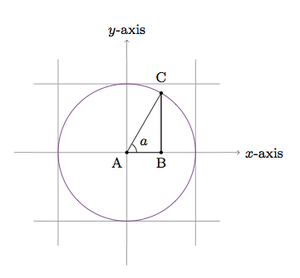# Calculations with sine and cosine

Alignments to Content Standards: F-TF.C.8

1. Complete the following table, rounding off each answer to the nearest hundredth if using a calculator. Draw a picture showing the quantities $\sin{x}$ and $\cos{x}$ calculated in the table.

Angle (degrees) $\cos{x}$ $\sin{x}$ $\cos{x} + \sin{x}$ $\cos^2{x} + \sin^2{x}$
$0$
$15$
$30$
$45$
$60$
$75$
$90$
2. What patterns do you notice in the second and third columns? Do these patterns hold for other angle measures as well? Explain.

3. What do you notice about the numbers in the fourth column of the table?  When does it $\cos{x} + \sin {x}$ appear to take its maximal value?
4. What do you notice about the numbers in the fifth column of the table? Will this hold for other angle measures? Explain.

## IM Commentary

The goal of this task is to study some values of $\sin{x}$ and $\cos{x}$ looking for and then explaining patterns. The teacher may wish to give more angles (including obtuse angles) in which case more patterns may become apparent. Students have many options for filling in the table:

1. using a calculator, as indicated,
2. using graph paper and a protractor to estimate the coordinates of points on the unit circle,
3. using trigonometric addition formulas plus knowedge of $\sin{x}$ and $\cos{x}$ for special angles (30 degrees, 45 degrees, and 60 degrees).

If students use method (a) or (b) then their values for $\sin^2{x} + \cos^2{x}$ may be very close to but not equal to 1.

The table below shows the exact values of $\sin{x}$ and $\cos{x}$ for the given angles:

Angle $\cos{x}$ $\sin{x}$
$0$ 1 0
$15$ $\frac{\sqrt{6}+\sqrt{2}}{4}$ $\frac{\sqrt{6}-\sqrt{2}}{4}$
$30$ $\frac{\sqrt{3}}{2}$ $\frac{1}{2}$
$45$ $\frac{\sqrt{2}}{2}$ $\frac{\sqrt{2}}{2}$
$60$ $\frac{1}{2}$ $\frac{\sqrt{3}}{2}$
$75$ $\frac{\sqrt{6}-\sqrt{2}}{4}$ $\frac{\sqrt{6}+\sqrt{2}}{4}$
$90$ 0 1

A complete argument that the value of $\sin(x)+\cos(x)$ peaks at $\frac{\sqrt{2}}{2}$ when $x=45^\circ$ is not included in this task, but there are some very elegant solutions using geometry or trigonometric identities and this is further developed in the following task:  https://www.illustrativemathematics.org/illustrations/1894.

## Solution

1. The table below was completed with a calculator:

Angle $\cos{x}$ $\sin{x}$ $\cos{x} + \sin{x}$ $\cos^2{x} + \sin^2{x}$
$0$ 1 0 1 1
$15$ 0.97 0.26 1.22 1
$30$ 0.87 0.5 1.37 1
$45$ 0.71 0.71 1.41 1
$60$ 0.5 0.87 1.37 1
$75$ 0.26 0.97 1.22 1
$90$ 0 1 1 1

In the table we have used a calculator and rounded all numbers to the nearest hundredth. Notice that the sum of the rounded sine and cosine values is not always the same as the rounded value of the sum (this fails for the 15, 45, and 75 degree angles). For the last column, a calculator will show that these values are very close to 1, as close as the calculator can find: the values are actually equal to 1 as will be deduced in part (d).

For an acute angle $a$, the values of $\sin{a}$ and $\cos{a}$ can be calculated using the following picture where the purple circle is the unit circle:Since the right triangle has its hypotenuse of unit length, the lengths of its legs are $\sin{a}$ and $\cos{a}$ as marked.

2. There are many patterns in the second and third columns. In the second column, the numbers decrease from 1 to 0 as the angle grows from 0 to 90. The third column, on the other hand, increases from 0 to 1 as the angle grows from 0 to 90 degrees.

Another interesting pattern is that the numbers in column 2 are the same as the numbers in column 3 listed in reverse order, that is $\cos{0} = \sin{90}$, $\cos{15} = \sin{75}$, and so on. More generally, for any acute angle $x$, $$\cos{x} = \sin{(90-x)}.$$ This can be explained via the following picture where the purple circle is the unit circle and the triangle is right:In the picture we see that $\sin{a} = |BC|$. Since $m(\angle B) = 90$ we have $m(\angle C) = 90 - a$. The picture also shows that $\cos{(90-a)} = |BC|$. This shows that $\sin{a} = \cos{(90-a)}$ for an acute angle measure $a$.

3. In the table, it appears as if $\sin{x} + \cos{x}$ might increase as $x$ increases from 0 to 45 and then decrease as $x$ goes from $45$ to $90$. This is consistent with the observation, made in part (b), that $\cos{x} + \sin{x} = \sin{(90-x) + \cos{(90-x)}}$.   More investigation is needed to see if and why this might be the case but further calculations for other angles will support this conjecture.  For example $\cos{35} + \sin{35}$ is about 1.39 and $\cos{40} + \sin{40}$ is a little less than 1.41 (whereas $\cos{45} + \sin{45}$ is a little larger than 1.41).

4. The numbers in the fifth column are all 1, suggesting the identity $$\cos^2{x} + \sin^2{x} = 1$$ when $0 \leq x \leq 90$. We can explain why this is true by further studying the geometry of right triangles. We return to the picture from part (a):The hypotenuse of the right triangle has length 1 as it lies on the unit circle. Therefore applying the Pythagorean theorem gives us $$\sin^2{a} + \cos^2{a} = 1$$ when $a$ is an acute angle.